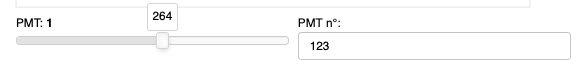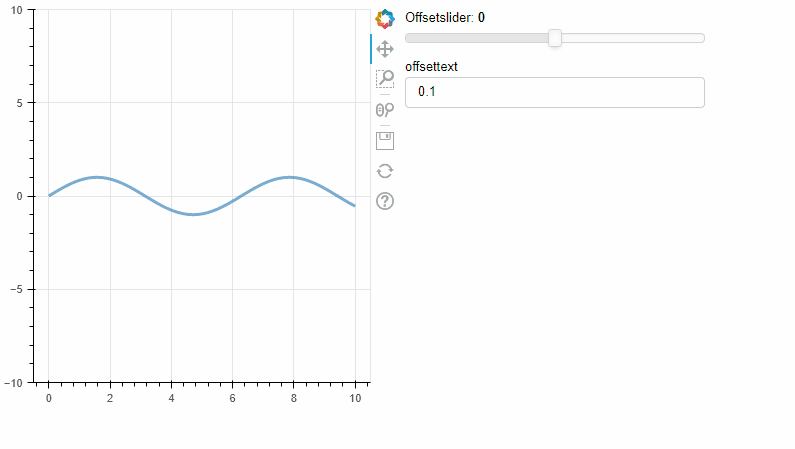# Slider + TextInput

Hi,

I use Slider and TextInput to modify the same value.
Is there a way to synchronize their values ?
When I modify one it will update automatically the other one.
In the following exampleI modify the slider value but the TextInput keep the previous value I have set .
Olivier

Hi @odadoun please provide the actual code you tried that didn’t work.

Hi @Bryan,

here the situation where I am …

``````import numpy as np
from bokeh.layouts import column, row
from bokeh.models import CustomJS, Slider
from bokeh.plotting import ColumnDataSource, figure, show
from bokeh.models.widgets import TextInput

x = np.linspace(0, 10, 500)
y = np.sin(x)

source = ColumnDataSource(data=dict(x=x, y=y))
plot = figure(y_range=(-10, 10), width=400, height=400)
plot.line('x', 'y', source=source, line_width=3, line_alpha=0.6)
offset_slider = Slider(start=-5, end=5, value=0, step=1, title="Offsetslider")
offset_text = TextInput(value="0.1", title="offsettext")

def thecallback(offset_type):
return CustomJS(args=dict(source=source, offset=offset_type),
code="""
const data = source.data;
const B = parseFloat(offset.value);
const x = data['x'];
const y = data['y'];
for (let i = 0; i < x.length; i++) {
y[i] = B + Math.sin(2*x[i]);
}
source.change.emit();
""")
offset_slider.js_on_change('value', thecallback(offset_slider))
offset_text.js_on_change('value', thecallback(offset_text))
layout = row(plot,column(offset_slider,offset_text))
show(layout)
``````

But I think I have took a wrong way …
cheers
Olivier

You’re pretty close.

The biggest thing is that you’re mixing up python-callbacks with CustomJS callbacks. With CustomJS, you don’t need to embed it in a python function, you just assign the callback to the widget via js_on_change. You kinda did half and half.

The second thing is the callback code itself → you are really trying to make the function work for both cases: one) where user changes text input you want the slider to update, and two) where user changes slider you want the text to update. That’s totally possible to do (and my solution below does that), but you COULD simplify things by creating two callbacks, one to execute when the slider changes, and one to execute when the text input changes.

The tricky part with making it “one callback” is that you need a way to identify what widget was changed, and then update the other one. My solution is to use/abuse the “name” attribute (which is available on all bokeh models AFAIK).

``````import numpy as np
from bokeh.layouts import column, row
from bokeh.models import CustomJS, Slider
from bokeh.plotting import ColumnDataSource, figure, show
from bokeh.models.widgets import TextInput

x = np.linspace(0, 10, 500)
y = np.sin(x)

source = ColumnDataSource(data=dict(x=x, y=y))
plot = figure(y_range=(-10, 10), width=400, height=400)
plot.line('x', 'y', source=source, line_width=3, line_alpha=0.6)

#adding "name" attributes to the models can really help identify them later... see thecallback
offset_slider = Slider(start=-5, end=5, value=0, step=1, title="Offsetslider",name='slideyboi')
offset_text = TextInput(value="0.1", title="offsettext",name='textyboi')

thecallback = CustomJS(args=dict(source=source,os=offset_slider,ot=offset_text),
code="""
const data = source.data;
//useful: built into CustomJS funcs is the cb_obj variable, which refers to the object that is being altered/used
//so we can figure out what's being altered (i.e the slider or the text) via the name attribute attached to cb_obj
console.log(cb_obj.name)
// now just your basic if statement to handle either case

if (cb_obj.name == 'slideyboi'){
ot.value = os.value.toString()}
else if (cb_obj.name =='textyboi'){
os.value = parseInt(ot.value)}
const B = parseFloat(os.value);
// everything below here is unaltered
const x = data['x'];
const y = data['y'];
for (let i = 0; i < x.length; i++) {
y[i] = B + Math.sin(2*x[i]);
}
source.change.emit();
""")

offset_slider.js_on_change('value', thecallback)
offset_text.js_on_change('value', thecallback)
layout = row(plot,column(offset_slider,offset_text))
show(layout)
``````3 Likes

Very beautifulI had missed the cb_obj variable .
Thank you so much for the solution and the explanationscheers
Olivier

2 Likes

Just as a tangential FYI, note that I recently updated all the examples in the user’s guide to not use the `source.change.emit()` function, and instead follow a pattern more like:

``````callback = CustomJS(args=dict(source=source), code="""
const f = cb_obj.value
const x = source.data.x
const y = Array.from(x, (x) => Math.pow(x, f))
source.data = { x, y }
""")
``````

Assigning a new value to `source.data` will ensure that any updates that need to happen, will happen. I would definitely recommend everyone adopt this simpler / higher-level approach in new code.

1 Like

Hi,
thanks for this precision .
Need to apply this for a lot of .py filesThis topic was automatically closed 90 days after the last reply. New replies are no longer allowed.